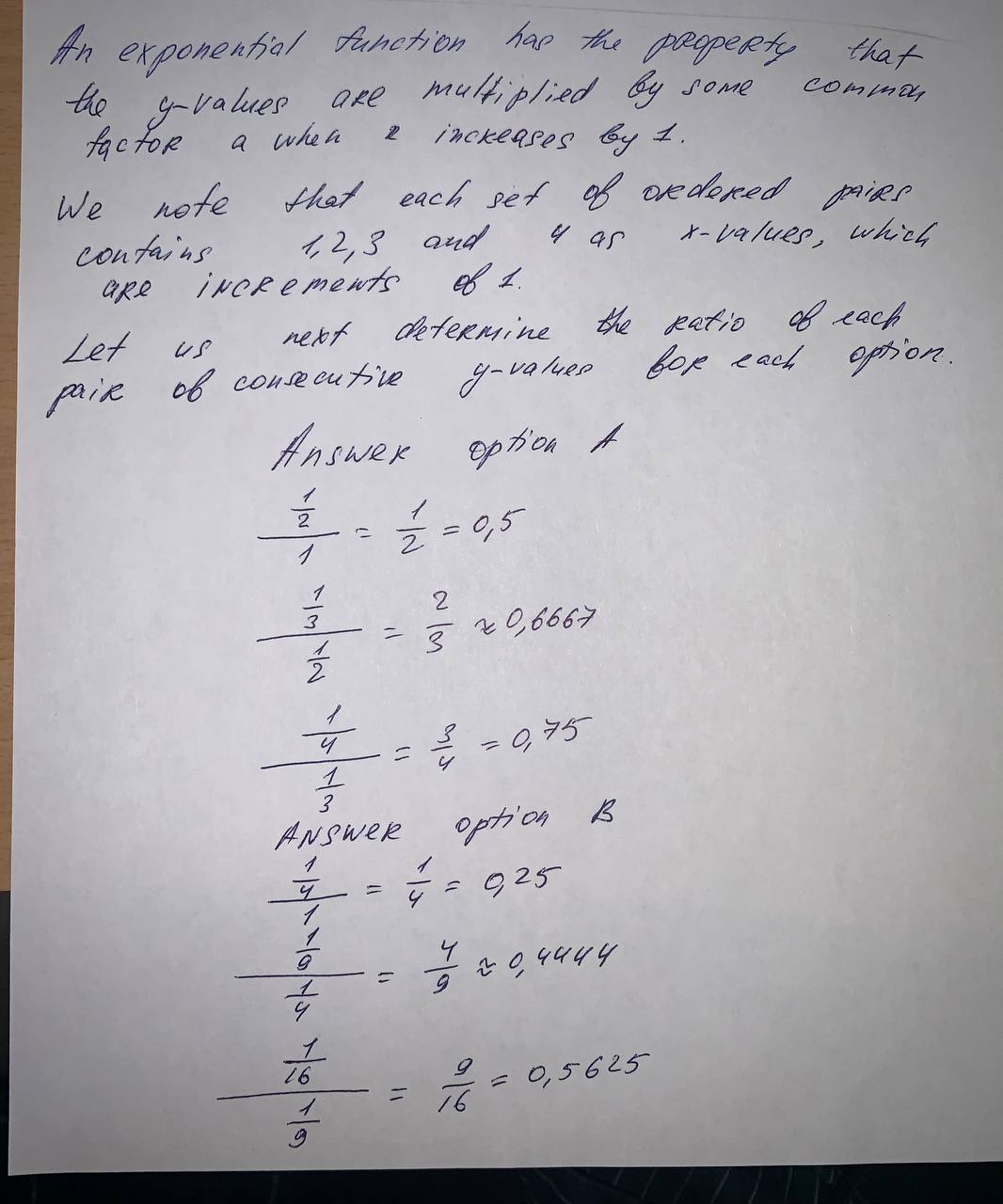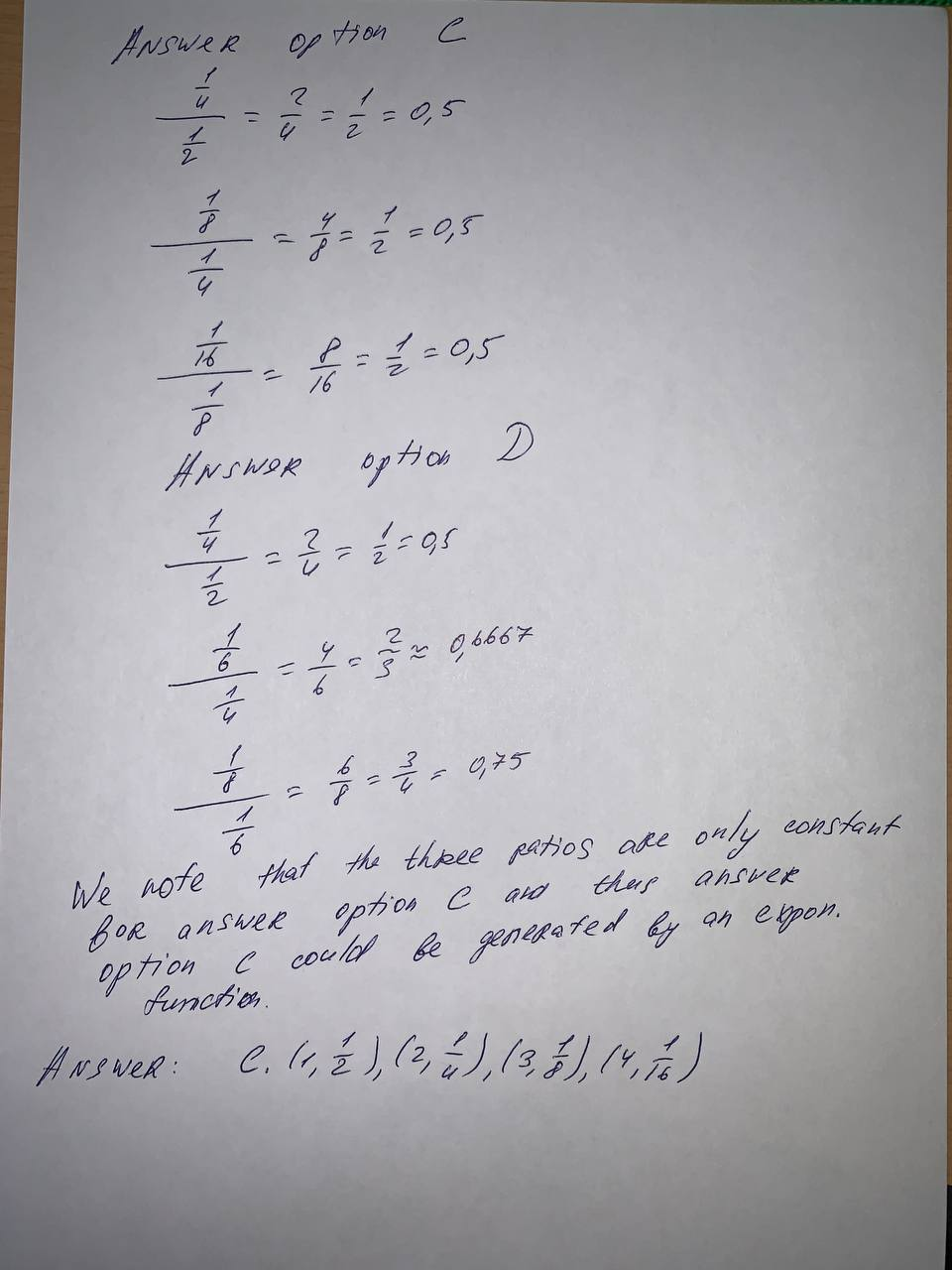# Which set of ordered pairs could be generated by anpedzenekO 2021-08-15 Answered
Which set of ordered pairs could be generated by an exponential function?
A. $\left(1,1\right)\left(2,\frac{1}{2}\right)\left(3,\frac{1}{3}\right)\left(4,\frac{1}{4}\right)$
B. $\left(1,1\right)\left(2,\frac{1}{4}\right)\left(3,\frac{1}{9}\right)\left(4,\frac{1}{16}\right)$
C. $\left(1,\frac{1}{2}\right)\left(2,\frac{1}{4}\right)\left(3,\frac{1}{8}\right)\left(4,\frac{1}{16}\right)$
D. $\left(1,\frac{1}{2}\right)\left(2,\frac{1}{4}\right)\left(3,\frac{1}{6}\right)\left(4,\frac{1}{8}\right)$
You can still ask an expert for help

• Questions are typically answered in as fast as 30 minutes

Solve your problem for the price of one coffee

• Math expert for every subject
• Pay only if we can solve iti1ziZ

Solution is given below